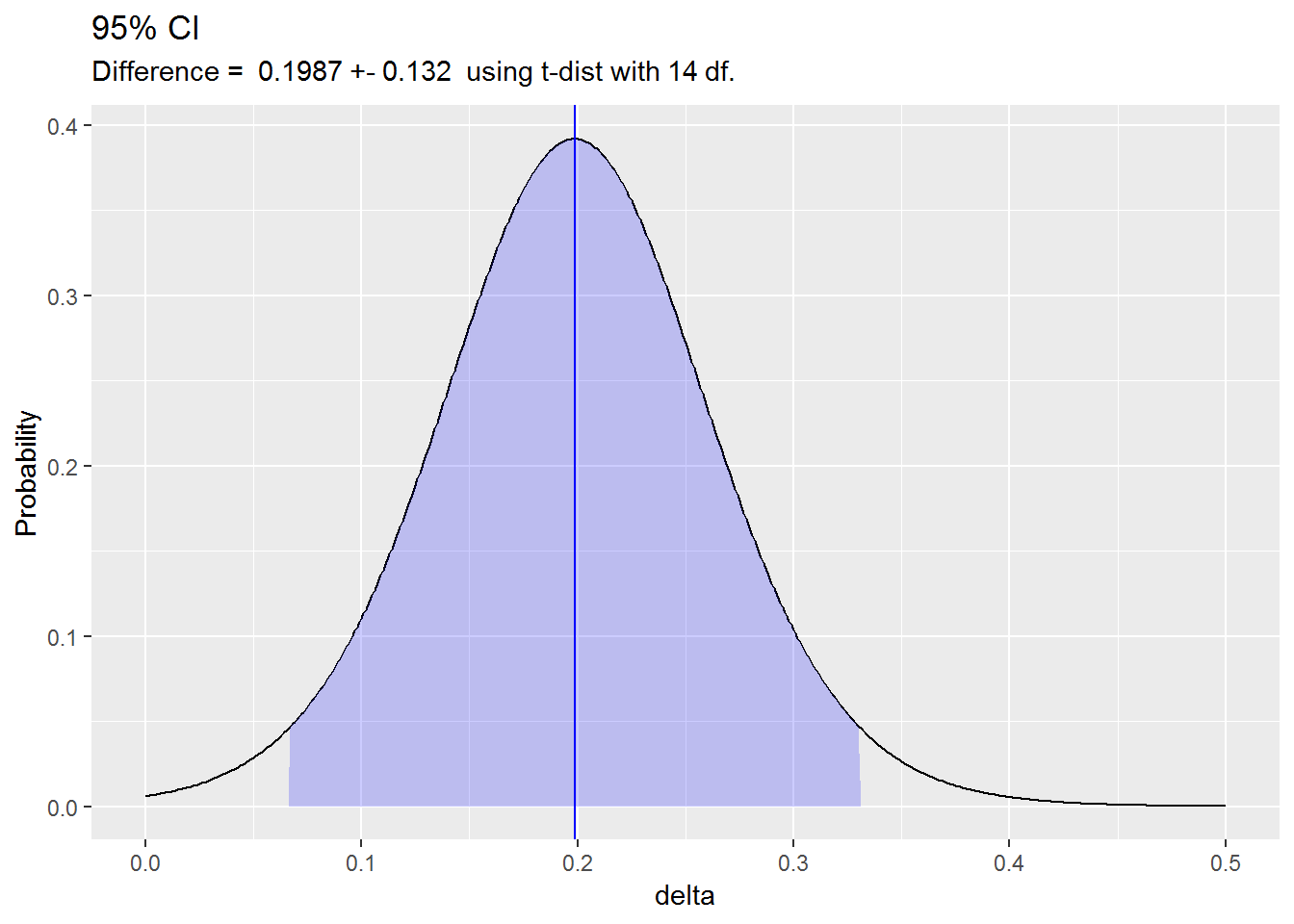The difference between independent means test uses the difference in sample means $$\hat{d} = \bar{x} - \bar{y}$$ as an estimate of the difference in population means $$d = \mu_X - \mu_Y$$ to evaluate an hypothesized difference in population means $$d_0$$. The null hypothesis is $$H_0: d = d_0$$. Alternatively, construct a $$(1 - \alpha)\%$$ confidence interval around $$\hat{d}$$ to estimate $$d$$ within a margin of error $$\epsilon$$.

In theory, you can test the difference between independent means with either a z test or a t test. Both require that the independent samples. Both require normal sampling distributions. The sampling distributions are normal if the underlying populations are normally distributed, or if the sample sizes are large $$(n >= 30)$$. However, the z-test additionally requires known sampling distribution variances $$\sigma^2_X$$ and $$\sigma^2_Y$$. These variances are never known, so always use the t-test.

In the z-test, the measured difference in means $$\hat{d} = \bar{x} - \bar{y}$$ approximates the difference in population means $$d = \mu_X - \mu_Y$$, and the sampling distribution of $$d$$ has a normal distribution centered at $$\hat{d} = d$$ with standard error $$se = \frac{\sigma_X}{\sqrt{n_X}} + \frac{\sigma_Y}{\sqrt{n_Y}}$$ where $$\sigma_X$$ and $$\sigma_Y$$ are the standard deviations of the underlying populations. Test $$H_0: d = d_0$$ with test statistic $$Z = \frac{\hat{d} - d_0}{se}$$ or define a $$(1 - \alpha)\%$$ confidence interval as $$d = \hat{d} \pm z_{1 - \alpha {/} 2} se$$.

The t-test is similar except that it estimates $$\sigma_X$$ and $$\sigma_Y$$ with the sample standard deviations $$s_X$$ and $$s_Y$$. The resulting sampling distribution has a t-distribution centered at $$\hat{d} = d$$ with standard error $$se = \frac{s_X}{\sqrt{n_X}} + \frac{s_Y}{\sqrt{n_Y}}$$. Test $$H_0: d = d_0$$ with test statistic $$T = \frac{\hat{d} - d_0}{se}$$, or define a $$(1 - \alpha)\%$$ confidence interval as $$d = \hat{d} \pm t_{1 - \alpha / 2, n_X + n_Y - 2} se$$.

#### Pooled vs Separate Variances

At this point in the t-test we have two options. If the sample sizes are small, and the standard deviations from each population are similar (roughly, $$s_X / s_Y$$ between $$0.5$$ and $$2$$), pool the sampling distribution variances $$s_p^2 = \frac{(n_X - 1) s_X^2 + (n_Y-1) s_Y^2}{n_X + n_Y-2}$$ so that $$se = s_p \sqrt{\frac{1}{n_X} + \frac{1}{n_Y}}$$. This version of the test is called the pooled variances t-test.

Otherwise let $$se = \sqrt{s_X^2 / n_X + s_Y^2 / n_Y}$$ and reduce the degrees of freedom in the t-distribution using Satterthwaite’s approximation $$df = \frac{(n_X - 1) (n_Y - 1)}{(n_Y - 1) C^2 + (1-C)^2 (n_X - 1)}$$ where $$C = \frac{s_X^2 / n_X}{se_\hat{d}^2}$$. This version of the t-test is called the separate variance t-test or Welch’s t-test.

#### Paired Samples

The paired two-sample test uses the mean of sampled paired differences $$\bar{d} = \sum_{i = 1}^n (x_i - y_i) {/} n$$ as an estimate of the mean of the population paired differences $$\delta$$ to evaluate the hypothesized mean of population paired differences $$\delta_0$$. Test $$H_0: \delta = \delta_0$$ with test statistic $$T = \frac{\bar{d} - \delta_0)}{se}$$, or define a $$(1 - \alpha)\%$$ confidence interval asw $$\delta = \bar{d} \pm t_{1 - \alpha / 2, n - 1} se$$. The paired t-test is really just a single mean t-test operating on variable that is defined as the difference between two variables.

## Pooled Variances t Test Example

A packing plant tests the hypothesis that a new machine is faster than its old machine. It measures the packing time for n_old = 10 cartons using the old new machine and n_new = 10 cartons using the new machine. At an $$\alpha = 5\%$$ level of significance, does the new machine pack faster?

library(readr)

machine <- read_tsv(file = "Data/machine.txt", col_names = TRUE)
colnames(machine)[colnames(machine) == "New machine"] <- "new"
colnames(machine)[colnames(machine) == "Old machine"] <- "old"

print(machine)
## # A tibble: 10 x 2
##      new   old
##    <dbl> <dbl>
##  1  42.1  42.7
##  2  41    43.6
##  3  41.3  43.8
##  4  41.8  43.3
##  5  42.4  42.5
##  6  42.8  43.5
##  7  43.2  43.1
##  8  42.3  41.7
##  9  41.8  44
## 10  42.7  44.1

The null hypothesis is that the difference in means is zero, $$H_0: d = \mu_{new} - \mu_{old} = 0$$ with alternative hypothesis that the new machine is faster, $$H_a: d < 0$$. The threshold level of significance s $$\alpha = 0.05$$.

d_0 = 0
alpha = 0.05

Prior to conducting a t test, check the initial conditions of indepependent samples and normally-distributed sampling distributions. The samples are independent because the machines are not related. The sample sizes are small (<30), so check that each sample is normally distributed. Neither normal Q-Q plot below indicates a non-normal population.

par(mfrow=c(1,2))
qqnorm(machine$old) qqline(machine$old)
qqnorm(machine$new) qqline(machine$new)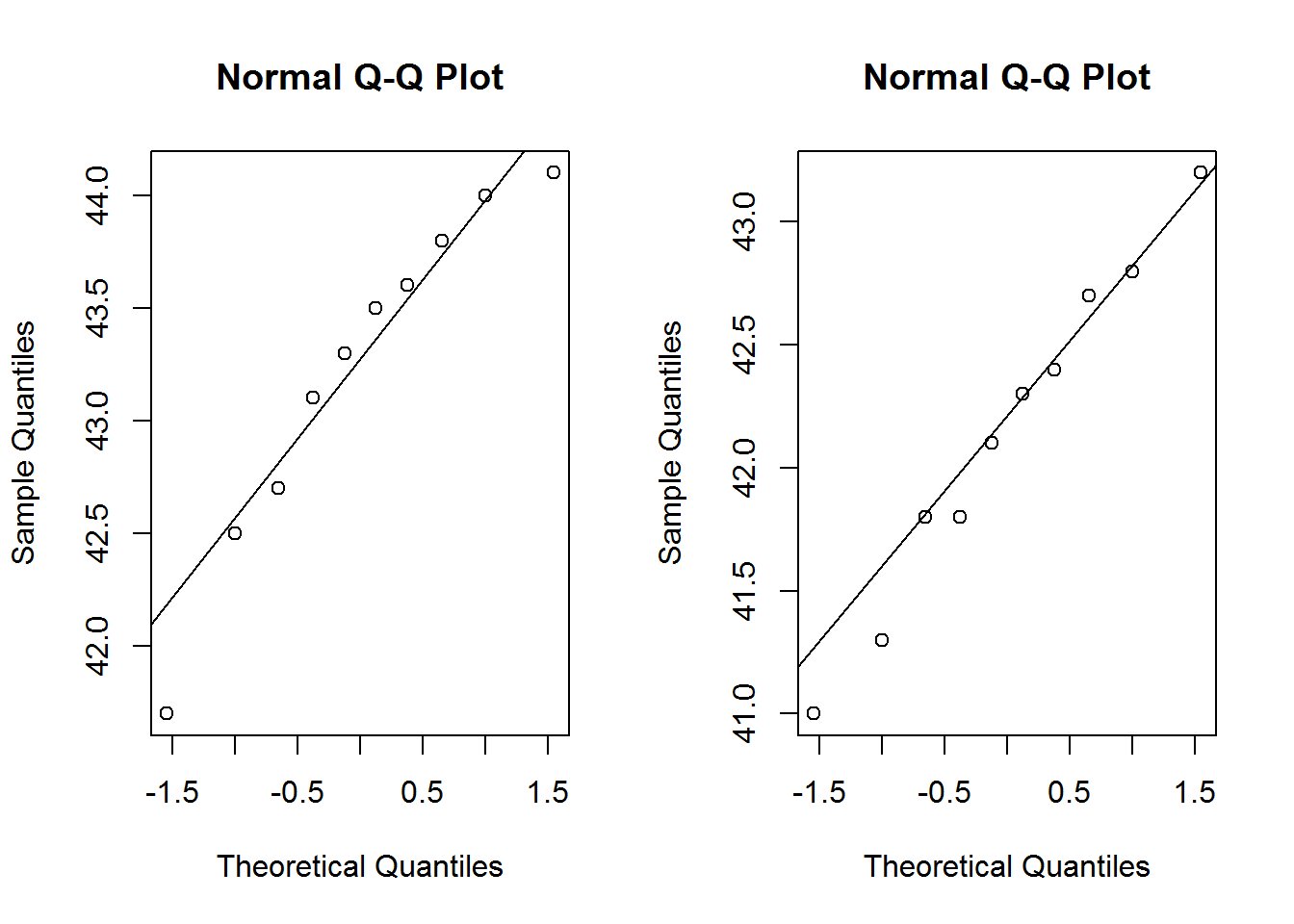The Anderson-Darling normality tests both have p-values > .05, so do not reject the null hypothesis of normally distributed populations.

library(nortest)
ad.test(machine$old) ## ## Anderson-Darling normality test ## ## data: machine$old
## A = 0.27475, p-value = 0.5782
ad.test(machine$new) ## ## Anderson-Darling normality test ## ## data: machine$new
## A = 0.14231, p-value = 0.9548

The pooled variance test can be used if the sample standard deviations are similar (their ratio between 0.5 and 2.0). That is the case here.

s_old = sd(machine$old) s_new = sd(machine$new)
s_old / s_new
##  1.097203

Better yet, use the F test to compare two variances. The F test compares uses the ratio of sample variances $$\hat{r} = s_X^2 / s_Y^2$$ as an estimate of the ratio of population variances $$r = \sigma_X^2 / \sigma_Y^2$$ to evaluate the hypothesized ratio of population variances $$r_0$$. In this case, $$H_0: r = \sigma_{new}^2 / \sigma_{old}^2 = 1$$. The p-value is $$P(f>|F|) = .7868$$, so do not reject the null hypothesis of equal variances in packing times.

r_0 = 1
alpha = 0.05
var.test(x = machine$new, y = machine$old,
ratio = 1,
alternative = "two.sided",
conf.level = .95)
##
##  F test to compare two variances
##
## data:  machine$new and machine$old
## F = 0.83067, num df = 9, denom df = 9, p-value = 0.7868
## alternative hypothesis: true ratio of variances is not equal to 1
## 95 percent confidence interval:
##  0.2063257 3.3442560
## sample estimates:
## ratio of variances
##          0.8306659

The pooled variance is $s_p^2 = \frac{(n_{new}-1) s_{new}^2 + (n_{old}-1) s_{old}^2)}{n_{new} + n_{old} - 2} = \frac{(10-1) .683^2 + (10-1) .750^2)}{10 + 10 - 2} = 0.515$.

n_old <- nrow(machine)
n_new <- nrow(machine)
(s_p2 <- ((n_new - 1) * s_new^2 + (n_old - 1) * s_old^2) /
(n_new + n_old - 2))
##  0.5147222

The variance of the measured difference sampling distribution is $se^2 = s_p^2 (\frac{1}{n_{new}} + \frac{1}{n_{old}}) = .515 (\frac{1}{10} + \frac{1}{10}) = .103$.

(se_2 <- s_p2 * (1 / n_new + 1 / n_old))
##  0.1029444

The test statistic for the pooled variance t-test for the difference in means is $T = \frac{\hat{d} - d_0}{\sqrt{se^2}} = \frac{(42.14 - 43.23) - 0}{\sqrt{0.103}} = -3.40$

d_hat <- mean(machine$new) - mean(machine$old)
(t <- (d_hat - d_0) / sqrt(se_2))
##  -3.397231

The p-value is $$P(t<T) = .0016$$, so reject the null hypothesis of no difference in packing times.

pt(q = t, df = n_new + n_old - 2, lower.tail = TRUE)
##  0.001605571

The function t.test() calculates the t-test for the difference in means. Specify var.equal = TRUE for a pooled variance.

(t.test.result <- t.test(x = machine$new, y = machine$old,
alternative = "less",
mu = 0,
paired = FALSE,
var.equal = TRUE,
conf.level = (1 - alpha)))
##
##  Two Sample t-test
##
## data:  machine$new and machine$old
## t = -3.3972, df = 18, p-value = 0.001606
## alternative hypothesis: true difference in means is less than 0
## 95 percent confidence interval:
##        -Inf -0.5336264
## sample estimates:
## mean of x mean of y
##     42.14     43.23

The plot of the hypothesis test shows the measured difference in means is well into the the range of rejection.

library(ggplot2)
library(dplyr)  # for %>%

t_crit = qt(p = alpha, df = n_old + n_new - 2)
lrr <- d_0 - t_crit * sqrt(se_2)
urr <- Inf
data.frame(d = -250:250 / 100) %>%
mutate(t = (d - d_0) / sqrt(se_2)) %>%
mutate(prob = dt(x = t, df = n_old + n_new - 2)) %>%
mutate(rr = ifelse(t < t_crit, prob, 0)) %>%
ggplot() +
geom_line(aes(x = d, y = prob)) +
geom_area(aes(x = d, y = rr), fill = "red", alpha = 0.3) +
geom_vline(aes(xintercept = d_0), color = "blue") +
geom_vline(aes(xintercept = d_hat), color = "red") +
labs(title = bquote("Hypothesis Test of H0: d = 0"),
subtitle = bquote("d_hat ="~.(round(d_hat, 1))~", p-value="~.(round(t.test.result$p.value, 4))), x = "d", y = "Probability") + theme(legend.position="none")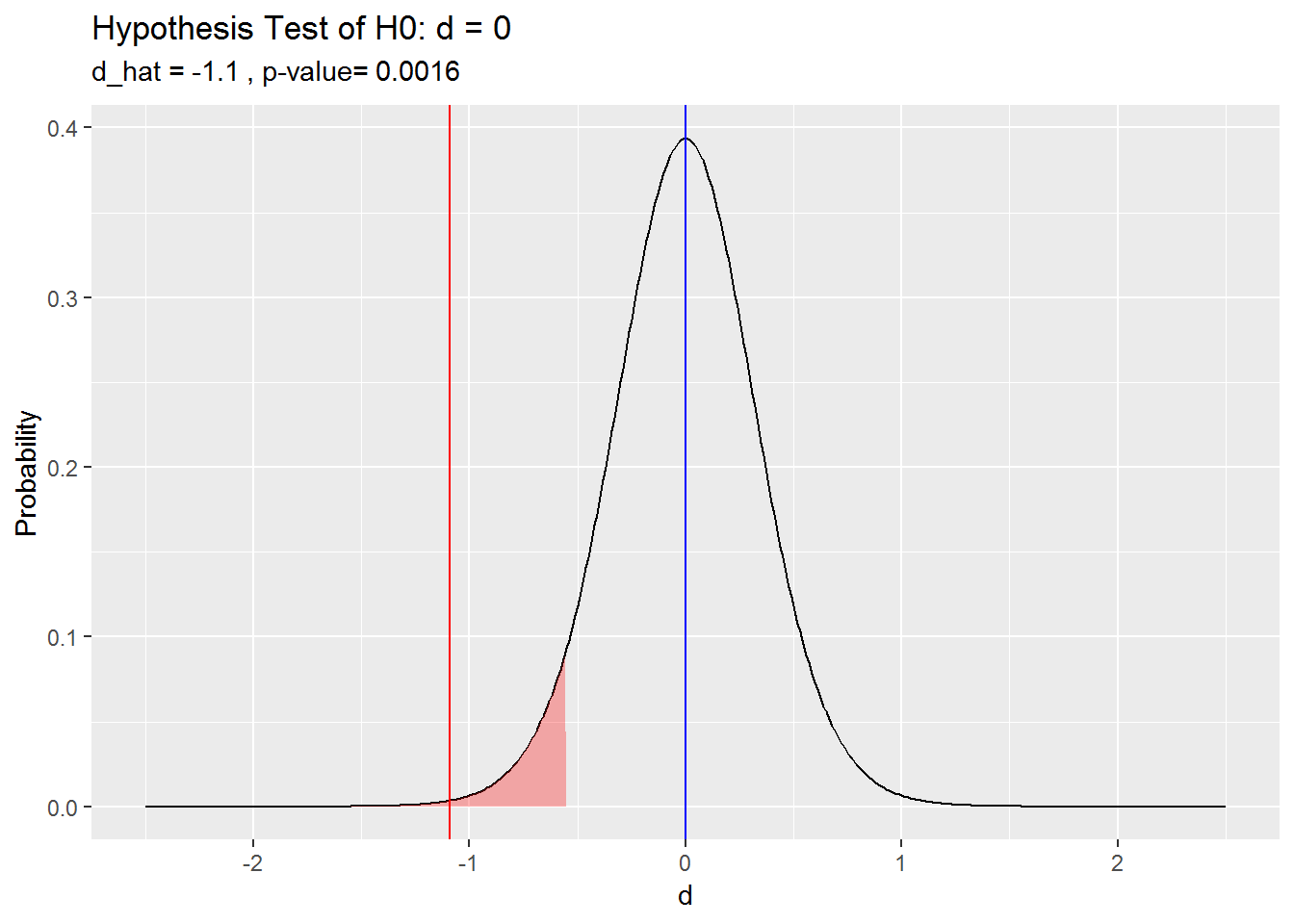Construct the pooled variance confidence interval for the difference in means. The confidence interval is $$d = \hat{d} \pm t_{\alpha / 2, n_X + n_Y - 2} se$$. t <- qt(p = alpha / 2, df = n_old + n_new - 2, lower.tail = FALSE) cat("95% CI: ", d_hat, " +/- ", t * sqrt(se_2), " (", d_hat - t * sqrt(se_2), ", ", d_hat + t * sqrt(se_2), ")") ## 95% CI: -1.09 +/- 0.6740799 ( -1.76408 , -0.4159201 ) The function t.test() calculates the t-test for the difference in means. Specify var.equal = TRUE for a pooled variance. (t.test.result <- t.test(x = machine$new,
y = machine$old, paired = FALSE, var.equal = TRUE, conf.level = (1 - alpha))) ## ## Two Sample t-test ## ## data: machine$new and machine$old ## t = -3.3972, df = 18, p-value = 0.003211 ## alternative hypothesis: true difference in means is not equal to 0 ## 95 percent confidence interval: ## -1.7640799 -0.4159201 ## sample estimates: ## mean of x mean of y ## 42.14 43.23 The plot of the confidence interval show the measured difference in means is significantly different from 0. library(ggplot2) library(dplyr) # for %>% t_crit = qt(p = alpha / 2, df = n_old + n_new - 2, lower.tail = FALSE) lcl <- -t_crit ucl <- t_crit data.frame(d = -250:250 / 100) %>% mutate(t = (d - d_hat) / sqrt(se_2)) %>% mutate(prob = dt(x = t, df = n_old + n_new - 2)) %>% mutate(rr = ifelse(t > lcl & t < ucl, prob, 0)) %>% ggplot() + geom_line(aes(x = d, y = prob)) + geom_area(aes(x = d, y = rr), fill = "blue", alpha = 0.1) + geom_vline(aes(xintercept = d_hat), color = "blue") + labs(title = bquote("95% CI for Difference in Means"), subtitle = bquote("d_hat = "~.(round(d_hat, 1))), x = "d", y = "Probability") + theme(legend.position="none")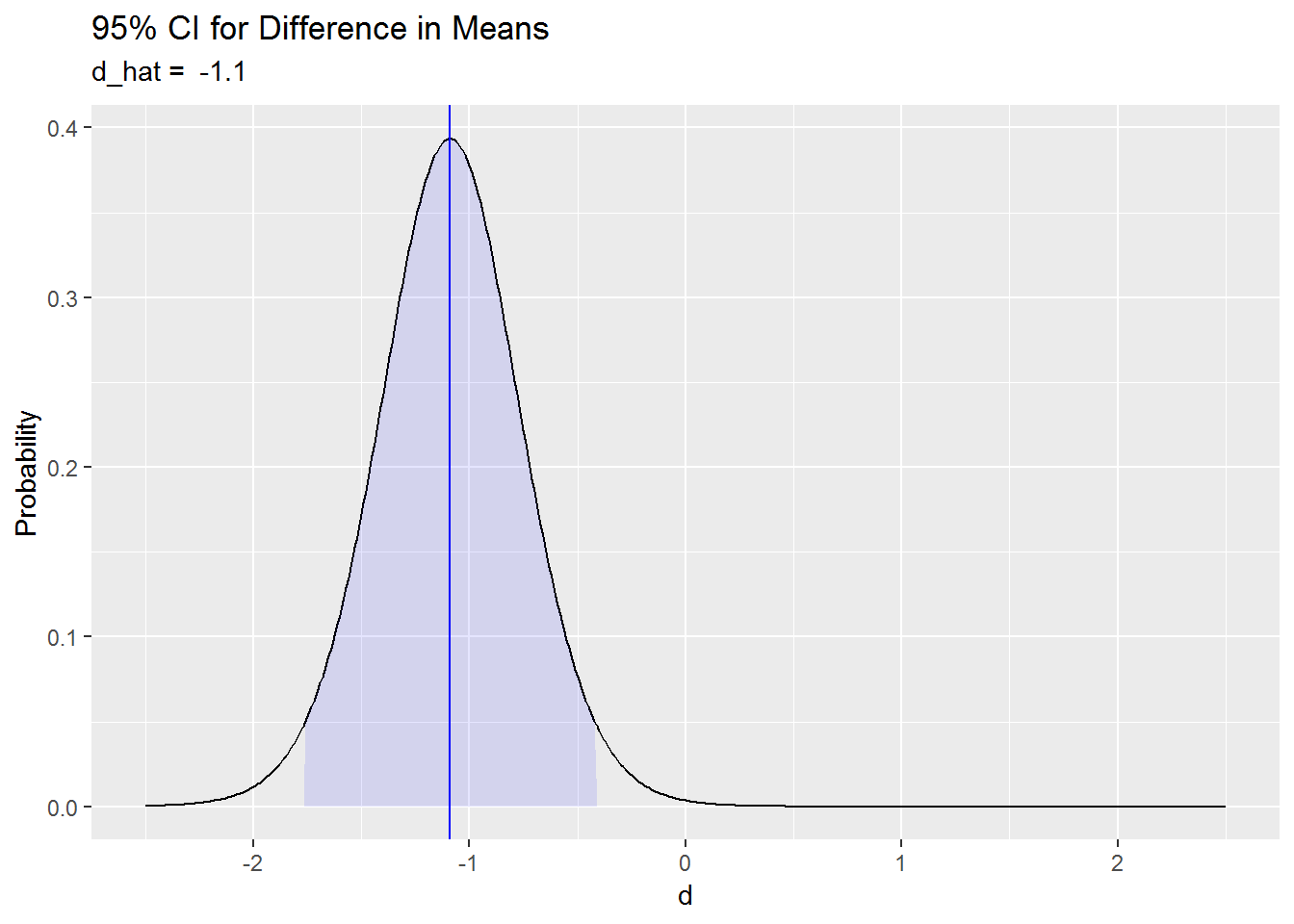### Example with separate Variances (Welch) In the example above, we could have used the separate variances t-test instead. How do the results differ? The separate variances method defines the standard error of the difference in means sampling distribution as $$se = \frac{s_{new}}{\sqrt{n_{new}}} + \frac{s_{old}}{\sqrt{n_{old}}} = \frac{0.6835}{\sqrt{10}} + \frac{0.7499}{\sqrt{10}} = 0.3208$$. The degrees of freedom are $$df = \frac{(n_new - 1) (n_old - 1)}{(n_old - 1) C^2 + (1-C)^2 (n_new - 1)} = \frac{(10 - 1) (10 - 1)}{(10 - 1) (.4537)^2 + (1 - .4537)^2 (10-1) } = 17.847$$ where $$C = \frac{s_{new}^2 {/} n_{new}}{se_\hat{d}^2} = \frac{(.6835)^2 {/} 10}{.3208^2} = 0.4537$$ (known as Satterthwaite’s approximation). (se <- sqrt(s_new^2 / n_new + {s_old}^2 / n_old)) ##  0.3208496 (c <- s_new^2 / n_new / se^2) ##  0.4537507 (df_satter <- (n_new - 1) * (n_old - 1) / ((n_old - 1) * c^2 + (1 - c)^2 * (n_new - 1))) ##  17.8473 Conduct the separate variance t-test for the difference in means. The T-statistic is $$T = \frac{\hat{d} - d_0}{\sqrt{se_\hat{d}^2}} = \frac{(42.14 - 43.23) - 0}{\sqrt{0.103}} = -3.40$$. The p-value is $$P(t<T) = .0016$$, so reject the null hypothesis of no difference in packing times. (t <- (d_hat - d_0) / sqrt(se)) ##  -1.924313 pt(q = t, df = df_satter, lower.tail = TRUE) ##  0.03520502 The stats package function t.test calculates the t-test for the difference in means. This time specify var.equal = FALSE for a separate variances test. (t.test.result <- t.test(x = machine$new,
y = machine$old, alternative = "less", mu = 0, paired = FALSE, var.equal = FALSE, conf.level = (1 - alpha))) ## ## Welch Two Sample t-test ## ## data: machine$new and machine\$old
## t = -3.3972, df = 17.847, p-value = 0.001621
## alternative hypothesis: true difference in means is less than 0
## 95 percent confidence interval:
##        -Inf -0.5333684
## sample estimates:
## mean of x mean of y
##     42.14     43.23

The separate variance method returned results nearly identical to the pooled variance method.

## Example with Paired Values

A study of 15 pairs of twins where one twin had schizophrenia measured the volumes of regions of the brain. What is the magnitude of the difference in volume?

x <- c(1.94, 1.44, 1.56, 1.58, 2.06,
1.66, 1.75, 1.77, 1.78, 1.92,
1.25, 1.93, 2.04, 1.62, 2.08)
y <- c(1.27, 1.63, 1.47, 1.39, 1.93,
1.26, 1.71, 1.67, 1.28, 1.85,
1.02, 1.34, 2.02, 1.59, 1.97)
d <- x - y
n <- length(d)

The null hypothesis is that the mean of the paired differences is zero, $$H_0: \delta = \delta_0 = 0$$ with alternative hypothesis that the difference is non-zero, $$H_a: \delta \ne 0$$. We use an $$\alpha = 0.05$$ level of significance.

d_0 <- 0
d_bar <- mean(d)
se <- sd(d) / sqrt(n)
alpha <- 0.05

The samples are independent because the sets of twins are not related. The sample size is not large $$(n < 30)$$, so we need to check that each population is normally distributed before taking a difference in pairs mean test with the t-statistic. Neither normal Q-Q plot below indicates a non-normal population. The Anderson-Darling normality tests have p-values > .05, so do not reject the null hypothesis of normally distributed populations.

qqnorm(x)
qqline(x)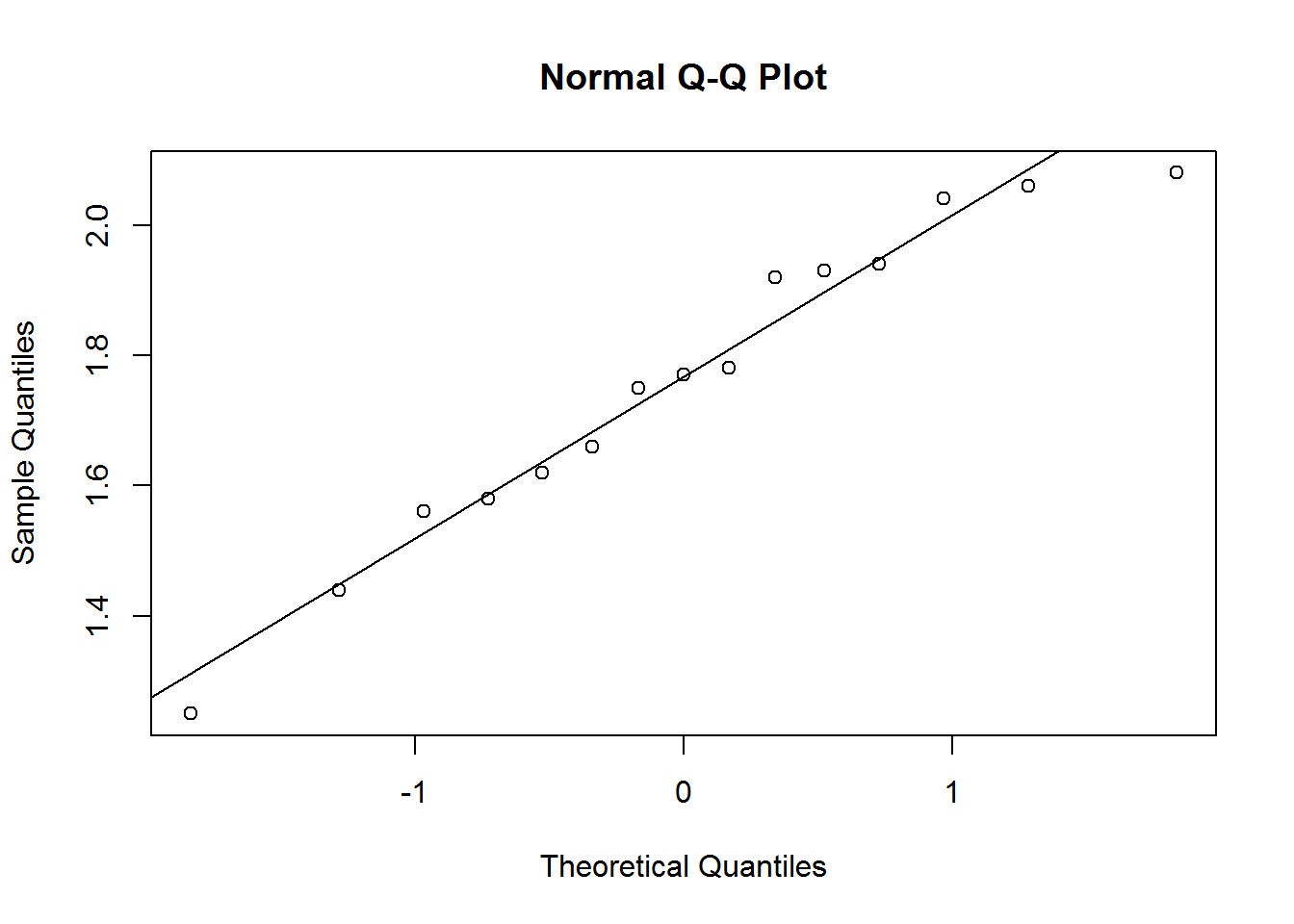qqnorm(y)
qqline(y)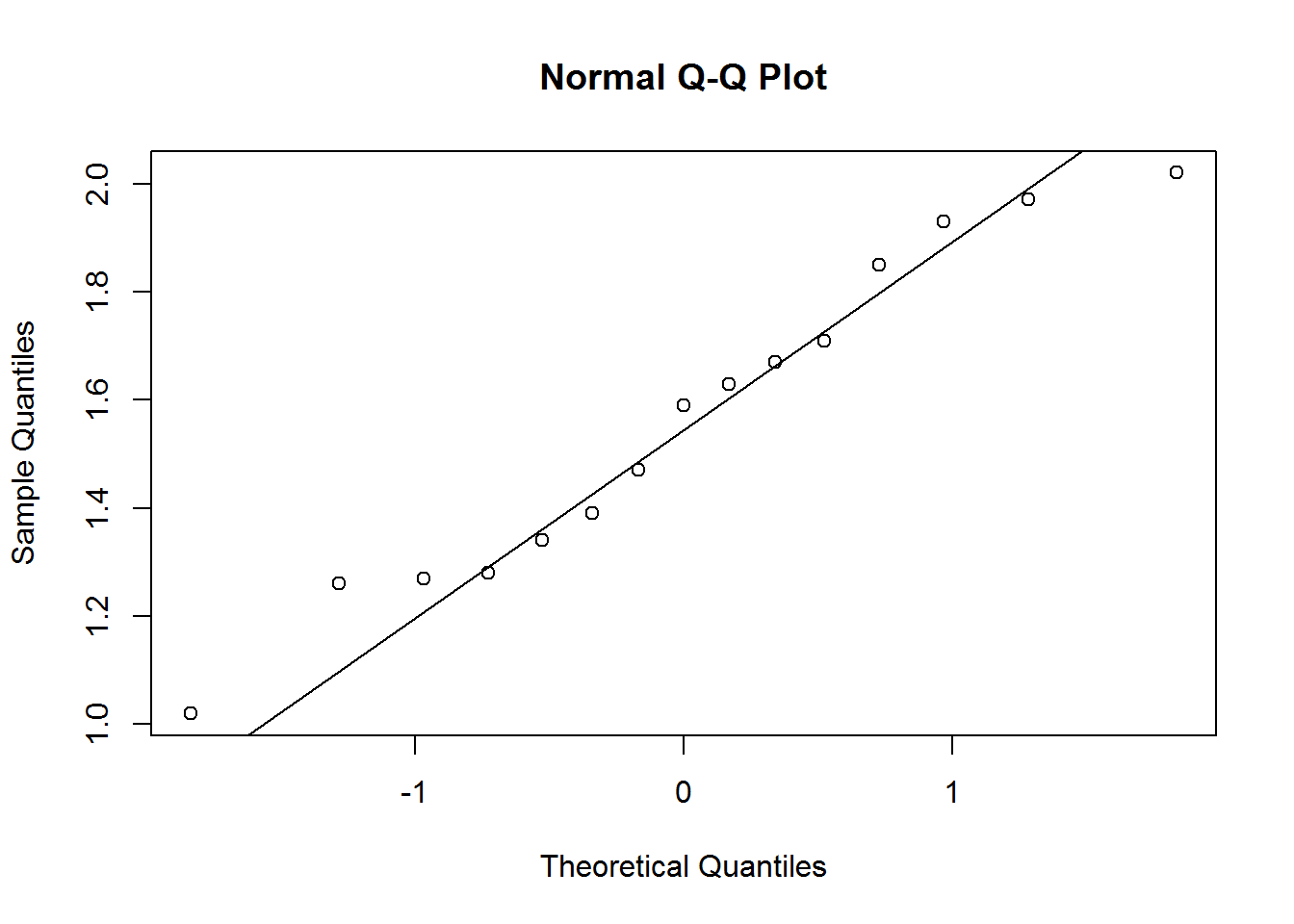library(nortest)
ad.test(x)
##
##  Anderson-Darling normality test
##
## data:  x
## A = 0.25027, p-value = 0.6937
ad.test(y)
##
##  Anderson-Darling normality test
##
## data:  y
## A = 0.25863, p-value = 0.6639

Conduct the t-test for the mean of paired differences. The T-statistic is $$T = \frac{\bar{d} - \delta_0)}{se} = \frac{0.1987 - 0}{0.0615} = 3.2289$$. The p-value is $$P(t<T) = .0061$$, so reject the null hypothesis of a mean difference of zero. Construct a 95% CI as $$d_bar \pm t_{1 - \alpha {/} 2, n - 1} se = .1987 \pm 0.1320 = (0.0667, 0.3306)$$.

(t <- (d_bar - d_0) / se)
##  3.228928
pt(q = t, df = n - 1, lower.tail = FALSE) * 2  # x2 for two-tail
##  0.006061544
(t_crit <- qt(p = alpha / 2, df = n - 1, lower.tail = FALSE))
##  2.144787
(epsilon <- t_crit * se)
##  0.1319626
(lcl <- d_bar - epsilon)
##  0.0667041
(ucl <- d_bar + epsilon)
##  0.3306292
cat("95% CI: (", lcl, ", ", ucl, ")")
## 95% CI: ( 0.0667041 ,  0.3306292 )

The R function t.test does these same calculations.

t.test(x = x,
y = y,
alternative = "two.sided",
mu = d_0,
paired = TRUE,
conf.level = (1 - alpha))
##
##  Paired t-test
##
## data:  x and y
## t = 3.2289, df = 14, p-value = 0.006062
## alternative hypothesis: true difference in means is not equal to 0
## 95 percent confidence interval:
##  0.0667041 0.3306292
## sample estimates:
## mean of the differences
##               0.1986667

Graph the t-test and CI.

# Hypothesis test graph
lrr <- d_0 - t * se
urr <- d_0 + t * se
data.frame(x = -500:500 / 1000) %>%
mutate(t = (x - d_0) / se) %>%
mutate(prob = dt(x = t, df = n - 1)) %>%
mutate(rr = ifelse(x < lrr | x > urr, prob, 0)) %>%
ggplot() +
geom_line(aes(x = x, y = prob)) +
geom_area(aes(x = x, y = rr), fill = "red", alpha = 0.3) +
geom_vline(aes(xintercept = d_0), color = "blue") +
geom_vline(aes(xintercept = d_bar), color = "red") +
labs(title = bquote('Hypothesis Test of H0: delta = 0'),
subtitle = bquote('Diff = '~.(round(d_bar, 4))),
x = "d",
y = "Probability") +
theme(legend.position="none")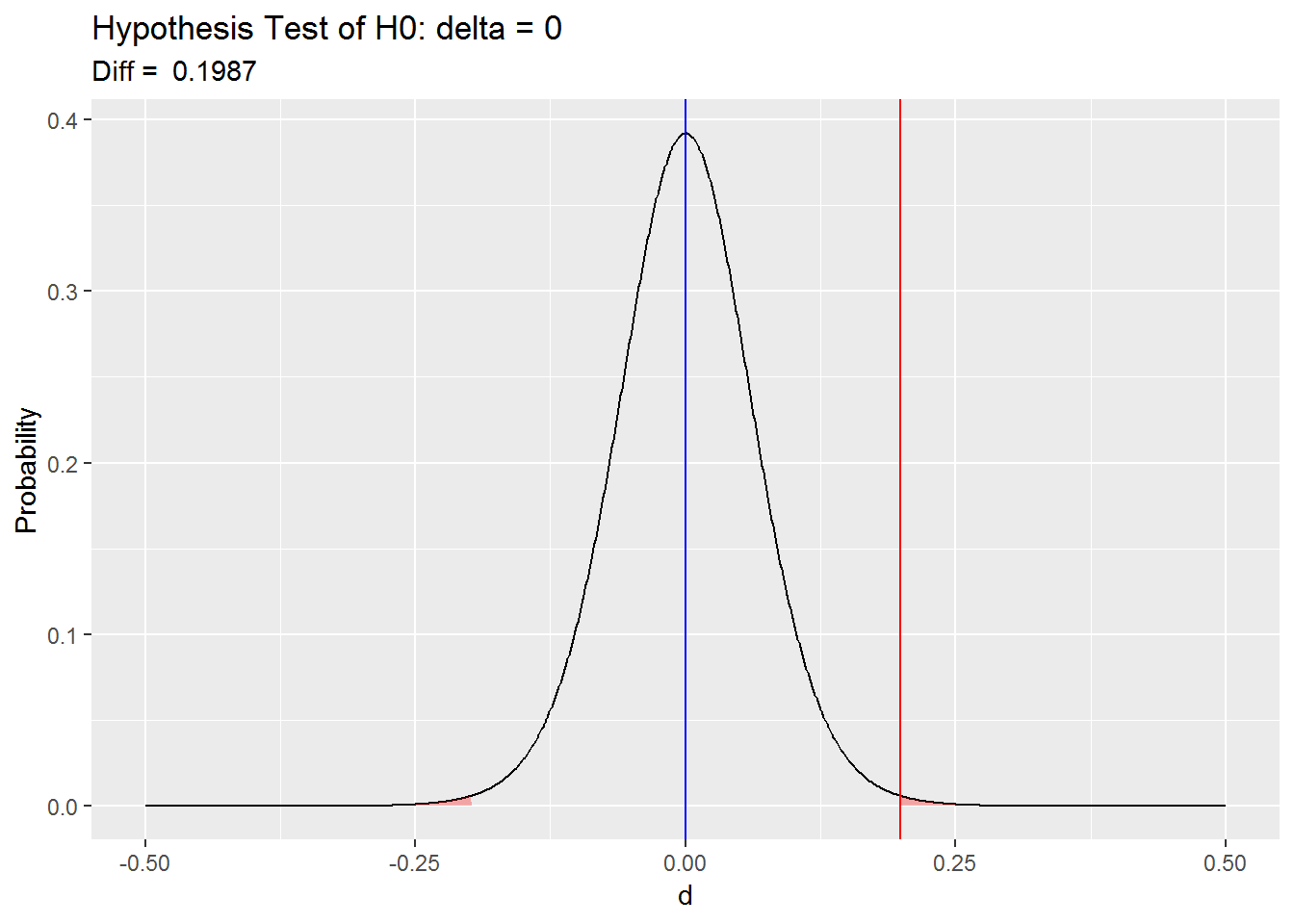# 95% confidence interval graph
data.frame(x = 0:500 / 1000) %>%
mutate(t = (x - d_bar) / se) %>%
mutate(prob = dt(x = t, df = n - 1)) %>%
mutate(rr = ifelse(x >= lcl & x <= ucl, prob, 0)) %>%
ggplot() +
geom_line(aes(x = x, y = prob)) +
geom_area(aes(x = x, y = rr), fill = "blue", alpha = 0.2) +
geom_vline(aes(xintercept = d_bar), color = "blue") +
labs(title = bquote('95% CI'),
subtitle = bquote('Difference = '~.(round(d_bar,4))~'+-'~.(round(epsilon, 4))~' using t-dist with'~.(n - 1)~'df.'),
x = "delta",
y = "Probability")